Warning

# Tutorial 6 - Phylogenetic trees - parsimony and probabilistic approaches

## Problem 1 - Fitch's algorithm

Using the Fitch algorithm, compute the total parsimony score under the Hamming distance and determine internal node labels.

How many different trees can be obtained?

## Problem 2 - Sankoff's algorithm (weighted parsimony)

As in the previous problem, the structure of the phylogenetic tree is already defined. Compute the total parsimony score using scoring matrix S and determine the internal nodes.

The scoring matrix $\mathbf{S}$ is defined as

\mathbf{S} = \begin{array}{c c} & \begin{array} {@{} c c c @{}} \hphantom{.}\mathrm{A}\hphantom{.} & \hphantom{.}\mathrm{C}\hphantom{.} & \hphantom{.}\mathrm{G}\hphantom{.} & \hphantom{.}\mathrm{T}\hphantom{.} \end{array} \\ \begin{array}{c} \mathrm{A} \\ \mathrm{C} \\ \mathrm{G} \\ \mathrm{T} \end{array}\hspace{-1em} & \left[ \begin{array}{@{} c c c @{}} 0 & 2.5 & 1 & 2.5 \\ 2.5 & 0 & 2.5 & 1 \\ 1 & 2.5 & 0 & 2.5 \\ 2.5 & 1 & 2.5 & 0 \end{array} \right] \\ \mbox{} % Blank line to match column names so as to align the = vertically \end{array}

and the structure of the tree is following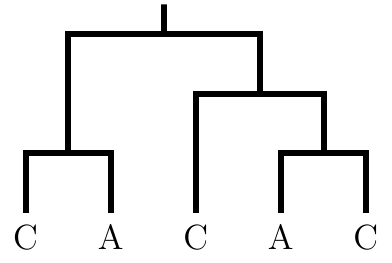How many different trees can be obtained?
What is the relationship between Fitch and Sankoff algorithm?

## Problem 3 - Felsenstein's algorithm

Assume that the Jukes-Cantor model holds, i.e., the probability of substitutions can be expressed by the following matrix

\begin{array}{c c} & \begin{array} {@{} c c c @{}} \hphantom{.}\mathrm{A}\hphantom{.} & \hphantom{.}\mathrm{C}\hphantom{.} & \hphantom{.}\mathrm{G}\hphantom{.} & \hphantom{.}\mathrm{T}\hphantom{.} \end{array} \\ \begin{array}{c} \mathrm{A} \\ \mathrm{C} \\ \mathrm{G} \\ \mathrm{T} \end{array}\hspace{-1em} & \left[ \begin{array}{@{} c c c @{}} 0.7 & 0.1 & 0.1 & 0.1 \\ 0.1 & 0.7 & 0.1 & 0.1 \\ 0.1 & 0.1 & 0.7 & 0.1 \\ 0.1 & 0.1 & 0.1 & 0.7 \end{array} \right] \\ \mbox{} % Blank line to match column names so as to align the = vertically \end{array} and that all bases are equally likely in the tree root. What is the likelihood of the following tree assuming that all edges have unit length?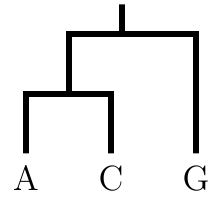## Problem 4 - Parsimony, likelihood, and bootstrapping

bash

wget https://cw.fel.cvut.cz/wiki/_media/courses/bin/tutorials/dnaprimates.txt
The file contains a selected block of mitochondrial DNA of several primates, including human (homo in Latin) and chimpanzee (pan in Latin). The file was published with the following paper:

• Maddison, D. R., Swofford, D. L., & Maddison, W. P. (1997). NEXUS: an extensible file format for systematic information. Systematic biology, 46(4), 590-621.

Now, switch to the R environment, and load the required libraries.

R

library("phangorn")
library("ape")

The following code loads the file with sequences.

R

primates = read.phyDat("dnaprimates.txt", format="phylip", type="DNA")

To solve the small parsimony problem, we need to build a phylogenetic tree. To do that, the distance matrix needs to be calculated.

R

dm = dist.dna(as.DNAbin(primates))
Now we can build a tree as in the last tutorial.

R

treeUPGMA = upgma(dm)
treeNJ = NJ(dm)
To view the trees, the following commands may be used.

R

plot(treeUPGMA, main="UPGMA", cex = 0.8)
plot(treeNJ, "unrooted", main="NJ", cex = 0.5)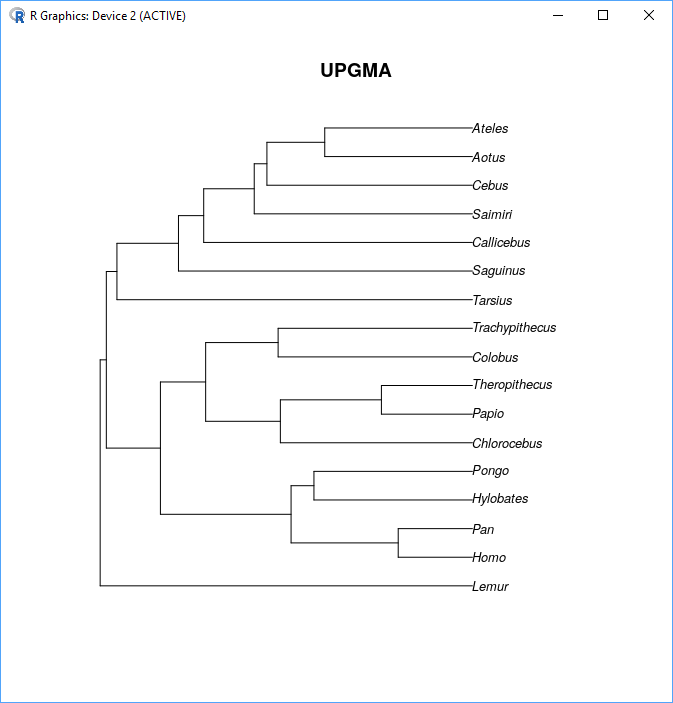### Parsimony score and the most parsimonious tree

To calculate the parsimony score, you can use the following commands.

R

parsimony(treeUPGMA, primates)
parsimony(treeNJ, primates)

Which tree is better? Why? Is it truly the most parsimonious tree?

Clearly, we can pick a better tree out of the two. However, we need to be sure to find the best one. To do that call

R

optParsUPGMA = optim.parsimony(treeUPGMA, primates)
optParsNJ = optim.parsimony(treeNJ, primates)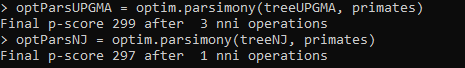And again, we can plot the trees by

R

plot(optParsUPGMA, main="UPGMA", cex = 0.8)
plot(optParsNJ, "unrooted", main="NJ", cex = 0.5)

### Maximum likelihood tree

Parsimony is not the only way how to evaluate a tree. We can calculate the probability of the tree given the data. The following code calculates the probability of the trees.

R

likeliNJ <- pml(treeNJ, primates)
print(likeliNJ)

likeliUPGMA <- pml(treeUPGMA, primates)
print(likeliUPGMA)
As in the last case, quality evaluation is good; however, we are interested in finding the optimal tree.

R

optLikeliNJ <- optim.pml(likeliNJ, model = "JC", rearrangement = "stochastic")
optLikeliUPGMA <- optim.pml(likeliUPGMA, model = "JC", rearrangement = "stochastic")
Parameter model = “JC” tells R to use the Jukes-Cantor model for scoring the tree. There are many other models; they differ in the number of free parameters in the matrix of substitution probabilities. You may replace Jukes-Cantor model TN93, which is named after Tamura-Nei and the year of publication.

Calling

R

logLik(optLikeliNJ)
logLik(optLikeliUPGMA)
shows that the tree likelihood improved.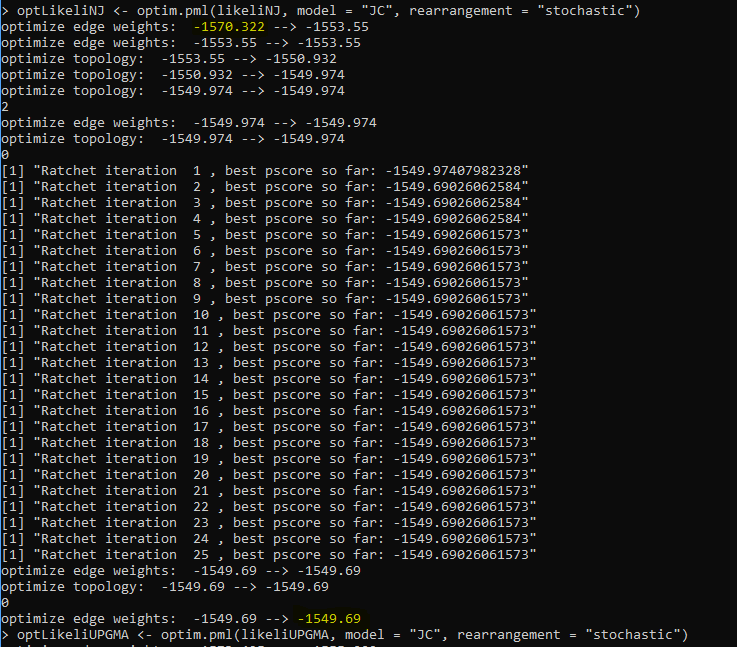### Bootstrapping

The last method that shows tree quality which we will mention today is different from the previous. The tree can be calculated if we omit some of the columns in all sequences. In other words, we sample the columns of the multiple-sequence alignment. In the next step, we can compare how often two nodes are in the same position in a tree after sampling and in the reference tree we evaluate. In R, the following single line command does the work for the neighbor-joining tree.

R

bs <- bootstrap.pml(optLikeliNJ, bs=100, optNni=TRUE, multicore=TRUE, control = pml.control(trace=0))
plotBS(midpoint(optLikeliNJ\$tree), bs, p = 50, type="p")
The result can be seen below. In how many cases were human and chimpanzee classified as closest relatives?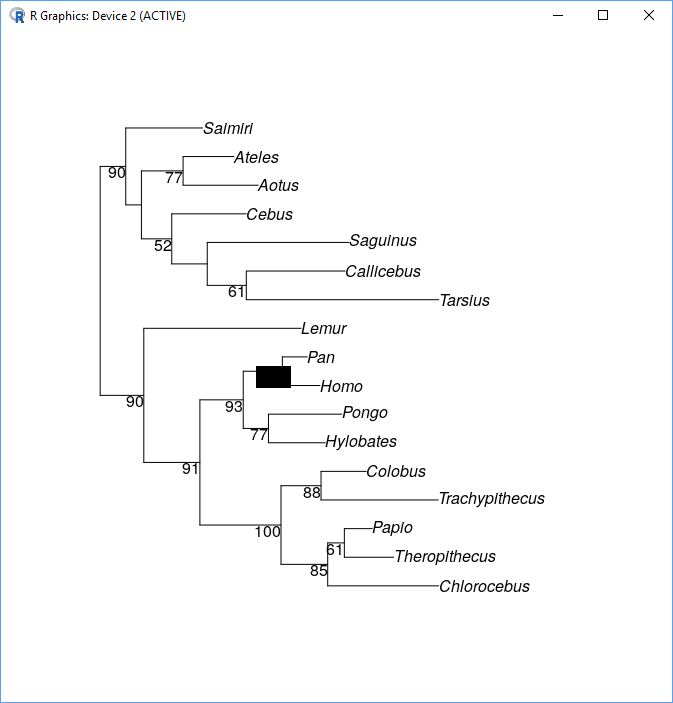This tutorial is a combination of the following: